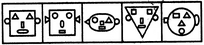Non Verbal Reasoning - Classification - Discussion

Discussion :: Classification - Section 2 (Q.No.42)

In each problem, out of the five figures marked (1), (2), (3), (4) and (5), four are similar in a certain manner. However, one figure is not like the other four. Choose the figure which is different from the rest.

42.

Choose the figure which is different from the rest.(1)     (2)     (3)     (4)     (5)

 [A]. 1 [B]. 2 [C]. 3 [D]. 4 [E]. 5

Explanation:

All other figures contain two circles, two triangles, one square, one rectangle and one ellipse while fig. (4) contains two squares.